# Quasi-linearization

(diff) ← Older revision | Latest revision (diff) | Newer revision → (diff)

A collection of methods for the numerical solution of non-linear problems by reducing them to a sequence of linear problems. Lying at the basis of the apparatus of quasi-linearization is the Newton method and its generalization to function spaces, the theory of differential inequalities (cf. Differential inequality) and the method of dynamic programming. The simplest example illustrating a method of quasi-linearization is the use of the Newton–Raphson method for finding the rootof a scalar monotone-decreasing strictly-convex function. In case the original non-linear functionis approximated at each stage of the iteration process by a linear function, the root ofis found, which serves as the next approximation, so thatUnder fairly general conditions the sequence so constructed has the property of monotonicity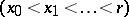and quadratic convergence: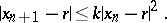The application of quasi-linearization to solving the Riccati equation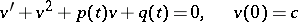(it is assumed that a solution exists on an interval), is as follows. The original equation is replaced by the equivalent equation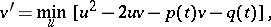where the minimum is taken over all functionsdefined on. This equation has a number of properties inherent to linear equations, and in order to solve it one uses the linear differential equation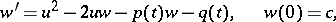whereis a fixed function. By appealing to the property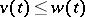(equality holding when), one can construct a system of successive approximationssatisfying the linear equations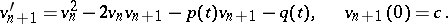The same recurrence relation can be obtained by applying the Newton–Kantorovich method (cf. Kantorovich process) to the original non-linear equation.

The employment of a quasi-linearization scheme in the solution of boundary value problems for the non-linear second-order differential equation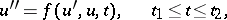leads to a sequence of functionssatisfying the linear equations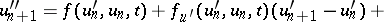with the linearized boundary conditions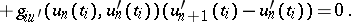The existence, uniqueness and quadratic convergence of the sequence follows from the corresponding convexity of the functions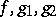over a sufficiently small interval.

The method of quasi-linearization finds application in the solution of two-point and multi-point boundary value problems for linear and non-linear ordinary differential equations, boundary value problems for elliptic and parabolic partial differential equations, variational problems, differential-difference and functional-differential equations, etc. As with every iteration scheme, the method of quasi-linearization is suitable for computer implementation and has various modifications enabling one to accelerate the convergence for narrower classes of problems. There exists a variety of examples of its use as a heuristic method for solving a number of problems in physics, technology and economy.

How to Cite This Entry:
Quasi-linearization. Encyclopedia of Mathematics. URL: http://encyclopediaofmath.org/index.php?title=Quasi-linearization&oldid=17538
This article was adapted from an original article by I.A. Vatel'F.I. Ereshko (originator), which appeared in Encyclopedia of Mathematics - ISBN 1402006098. See original article